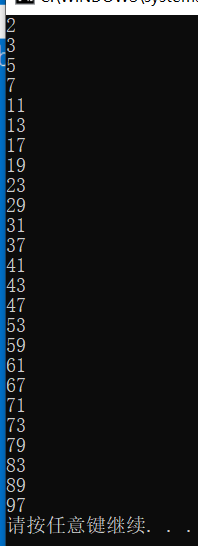# Java学习第二天2021.7.20

2021/7/20 19:31:41 浏览：

### Java

• 流程控制语句
• 顺序流程
• 条件控制
• 循环控制
• while
• do .... while
• for
• 双层循环
• 分支语句
• break
• continue
• return
• 练习题
• 找到偶数
• 水仙花数
• 九九乘法表
• 找到质数

# 流程控制语句

## 条件控制

``````class demo1
{
public static void main(String[] args){
int a = 3;
if(a == 3){
System.out.println("a的值和3相等");
}
}
}
``````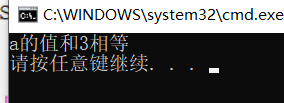``````class demo1
{
public static void main(String[] args){
int a = 4;
if(a == 3){
System.out.println("a的值和3相等");
}else{
System.out.println("a的值和3不相等");
}
}
}
``````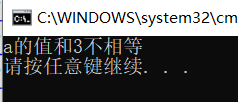``````class demo2
{
public static void main(String[] args){
int a = 60;
if(a<=100){
if(a>=80){
System.out.println("优秀");
}else if(a>=60){
System.out.println("良好");
}else{
System.out.println("不及格");
}
}
}
}
``````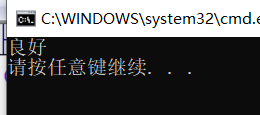## 循环控制

### while

``````class demo3
{
public static void main(String[] args){
int i = 0;
while(i<5){
System.out.println("hello world");
i++;
}
}
}
``````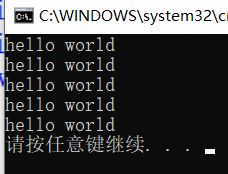### do … while

``````class demo4
{
public static void main(String[] args){
int i = 0;
do{
System.out.println("hello world");
i++;
}while(i<5);
}
}
``````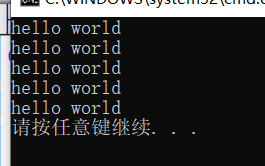``````class demo5
{
public static void main(String[] args){
int i = 0;
do{
System.out.println("hello world");
i++;
}while(i<0);
}
}
``````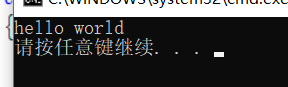### for

``````class demo6
{
public static void main(String[] args){
for(int i = 0;i < 5;i++){
System.out.println("hello world");
}
}
}
``````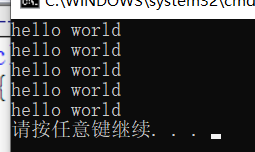### 双层循环

****
****
****
****

``````class demo7
{
public static void main(String[] args){
for(int i = 0;i<4;i++){
for(int j = 0;j<4;j++){
//print方法输出的时候不进行换行
System.out.print("*");
}
//这一行的意思是每次输出完一整行，然后要进行换行
System.out.println();
}
}
}
``````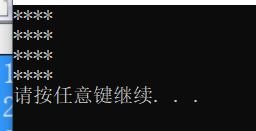1.创建一个空白项目并进行测试（不在演示）
2.先用四个语句进行输出一行数据

``````System.out.print("*");
System.out.print("*");
System.out.print("*");
System.out.print("*");
System.out.println();
``````

3.发现上面的代码有重复代码，然后可以用循环来改进一下

``````for(int j = 0;j<4;j++){
System.out.print("*");
}
System.out.println();
``````

4.这样就写完了一行的代码，然后输出四行，代码如下

``````for(int j = 0;j<4;j++){
System.out.print("*");
}
System.out.println();
for(int j = 0;j<4;j++){
System.out.print("*");
}
System.out.println();
for(int j = 0;j<4;j++){
System.out.print("*");
}
System.out.println();
for(int j = 0;j<4;j++){
System.out.print("*");
}
System.out.println();
``````

5.发现相同的代码又重复了，把3的代码当成一个整体放进另外一个循环中，让它循环执行里面的循环就行了，这样就出来了最后的代码

``````class demo7
{
public static void main(String[] args){
for(int i = 0;i<4;i++){
for(int j = 0;j<4;j++){
//print方法输出的时候不进行换行
System.out.print("*");
}
//这一行的意思是每次输出完一整行，然后要进行换行
System.out.println();
}
}
}

``````

## 分支语句

### break

``````class demo8
{
public static void main(String[] args){
for(int i = 0;i<10;i++){
if(i==5){
break;
}
System.out.println(i);
}
System.out.println("郑州在下雨");
}
}
``````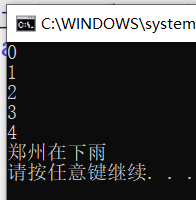### continue

``````class demo9
{
public static void main(String[] args){
for(int i = 0;i<10;i++){
if(i==5){
continue;
}
System.out.println(i);
}
System.out.println("郑州在下雨");
}
}
``````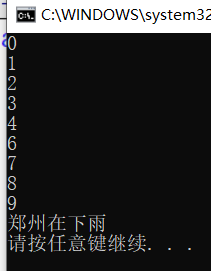### return

``````class demo10
{
public static void main(String[] args){
for(int i = 0;i<10;i++){
if(i==5){
return;
}
System.out.println(i);
}
System.out.println("郑州在下雨");
}
}
``````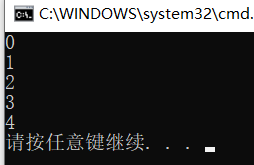# 练习题

## 找到偶数

1.先创建空白项目并进行测试
2.分析需求
3.先想办法把三位数全部都找到
4.把每个数字都进行对2求余，如果余数为0则为偶数，如果不为0则是奇数
5.定义两个变量，一个存储数字和，一个存储数字的个数

``````class demo011
{
public static void main(String[] args){
//先定义数字的总和sum并且初始化
int sum = 0;
//定义数字的个数
int n = 0;
//循环找出所有的三位数
for(int i = 100;i<1000;i++){
//对于i进行求余，如果余数为0则为偶数
if(i%2 == 0){
//如果是偶数，则会进入此代码块，sum+=i是sum = sum + i的另一种写法
sum+=i;
//数字的个数也自增
n++;
}
}
//输出数字的总和
System.out.println(sum);
//输出数字的个数
System.out.println(n);
}
}
``````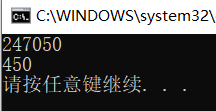## 水仙花数

1.先创建空白项目并进行测试
2.需求分析
3.先找出所有的三位数
4.把数字的三个位数上的数字拆开
5.把这三个数字的立方和与原来的数字进行比较
6.如果相同则进行输出

``````class demo021
{
public static void main(String[] args){
//这个用来存放个位数的数值
int g = 0;
//这个用来存放十位数的数值
int s = 0;
//这个用来存放百位数的数值
int b = 0;
//这个用来存放上面三个数字的立方和
int sum = 0;
//循环遍历所有的三位数字
for(int i = 100;i<1000;i++){
//提出来百位的数值
b = i / 100;
//提出来十位的数值
s = (i - b * 100)/10;
//提出来个位的数值
g = i - b * 100 - s * 10;
//算出来三个数字的立方和
sum = b*b*b + s*s*s + g*g*g;
//把sum和该数字进行比较
if(i==sum){
//相同则进行输出
System.out.println(i);
}
}
}
}
``````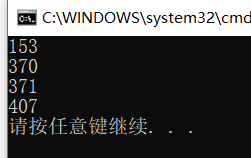## 九九乘法表

1.先创建一个空白项目并测试
2.分析这个需要嵌套循环来实现
3.外层的是右边的数字，内层循环是左边的数字
4.左边的数字需要小于等于右边的数字

``````class demo041
{
public static void main(String[] args){
//这个是外层循环，控制右边的数字
for(int i = 1;i<10;i++){
//这个是内层循环，控制的左边的数字
for(int j = 1;j<=i;j++){
//输出一个式子，不换行的
System.out.print(j+"*"+i+"="+j*i+" ");
}
//一行输出完了之后在进行换行
System.out.println();
}
}
}
``````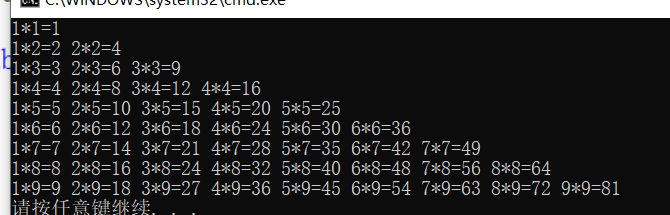## 找到质数

1.创建一个空白项目并进行测试
2.先遍历出2-100的所有数字
3.分析一下
4.质数的定义是要除了1和它本身都不能除尽，意思就是求余的结果不为0
5.可以定义一个开关来表示输出这个数字
6.在循环中写一个条件语句，如果求余结果为0，则开关变为false

``````class demo031
{
public static void main(String[] args){
//遍历2-100的数字
for(int i = 2;i<100;i++){
//定义一个锁，开始为true
int jishu = true;
//遍历2-i的数字来让i求余
for(int j = 2;j<i;j++){
//如果i%j为0，则表示i不是质数
if(i%j==0){
//锁变为false
jishu = false;
}
}
//如果jishu为true，则表示除了1和它本身没有能除尽的，为质数
if(jishu){
//输出这个质数
System.out.println(i);
}
}
}
}
``````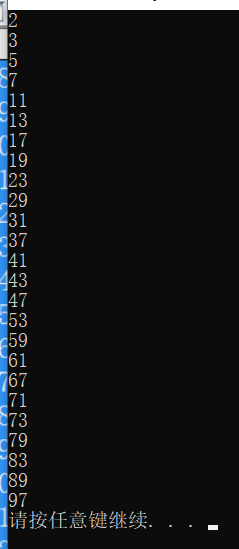``````import java.util.ArrayList;
class demo08
{
public static void main(String[] args){
ArrayList<Integer> arr = new ArrayList();
for(int i = 2;i<101;i++){
boolean suo = true;
for(int j = 0;j<arr.size();j++){
if(i % arr.get(j)==0){
suo = false;
break;
}
}
if(suo){
}
}
for(int i = 0;i<arr.size();i++){
System.out.println(arr.get(i));
}
}
}
``````